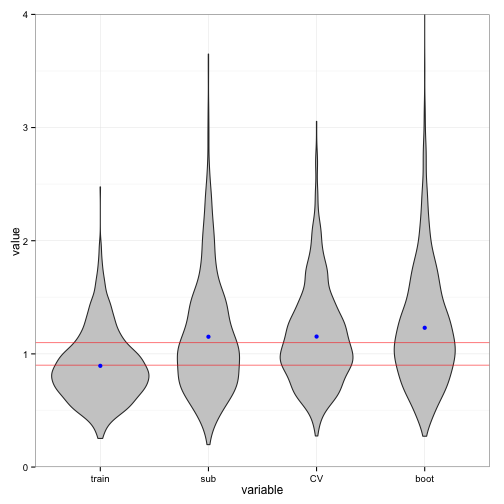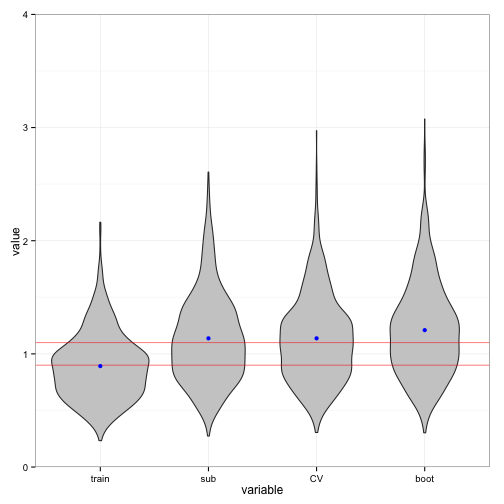# Estimation of the expected prediction error

This is an extended version of the R code presented in the lecture Friday, May 23, 2014. It contains an implementation and investigation of the three different simulation based methods for estimation of the expected prediction error.

## General functions

We first implement a number of generic functions for computing training error and estimates of expected prediction error by different methods.

trainErr <- function(form = y ~ x, data) {
model <- lm(form, data = data)
mean((data$y - predict(model))^2) }  Function for computing an estimate of expected prediction error based on subsampling. testSub <- function(form, data, B = 200, q = 0.75) { n <- nrow(data) PEsub <- vector("list", B) for(b in 1:B) { ## Subsampling a data set of size q * n i <- sample(n, floor(q * n)) modelsub <- lm(form, data = data[i, ]) muhat <- predict(modelsub, newdata = data[-i, ]) PEsub[[b]] <- (data$y[-i] - muhat)^2
}
mean(unlist(PEsub))
}


Function for computing an estimate of expected prediction error based on cross-validation.

testCV <- function(form, data, B = 50, k = 4) {
n <- nrow(data)
PEcv <- vector("list", B)
tmp <- numeric(n)
for(b in 1:B) {
## Generating the random subdivision into groups
group <- sample(rep(1:k, length.out = n))
for(i in 1:k) {
modelsub <- lm(form, data = data[group != i, ])
muhat <- predict(modelsub, newdata = data[group == i, ])
tmp[group == i] <- (data$y[group == i] - muhat)^2 } PEcv[[b]] <- tmp } mean(unlist(PEcv)) }  Function for computing an estimate of expected prediction error based on bootstrapping. testBoot <- function(form, data, B = 200) { n <- nrow(data) PEboot <- vector("list", B) for(b in 1:B) { i <- sample(n, n, replace = TRUE) modelsub <- lm(form, data = data[i, ]) muhat <- predict(modelsub, newdata = data[-i, ]) PEboot[[b]] <- (data$y[-i] - muhat)^2
}
mean(unlist(PEboot))
}


## First test example

First we apply the different functions on a single small simulated data set for a simple model where we just do linear regression. We simulate the data set and comute the training error and different estimates of expected prediction error.

n <- 20
x <- rnorm(n)
yx <- data.frame(y = rnorm(n, x), x)
form <- y ~ x
trainErr(form, yx)

##  1.041

testSub(form, yx)

##  1.317

testCV(form, yx)

##  1.368

testBoot(form, yx)

##  1.474


We know in this case that expected training error is $$1 - 2/n$$ (which is 0.9 when n = 20), and the expected prediction error is $$1 + 2/n$$ (which is 1.1 when n = 20). The subsampling and 4-fold cross-validation computations have mean $$1 + 2 / (qn)$$ – with $$q = 1 - 1/k$$ for $$k$$-fold CV. Thus in the case of $$q = 0.75$$ and $$n = 20$$ they have mean $$1.133$$. It is a little less clear what the theoretical mean for the bootstrap based estimates is. Note that the teoretical considerations are conditional on the predictors – this is reflected in the simulations below, where the predictors are simulated once and are common for the simulations of the responses.

## Simulation study

require(reshape2)
require(ggplot2)


In the simulation above we made a large number of replications (large $$B$$). In real examples this may be computationally prohibitive as this requires a large number of refits of the model. Here we investigate by simulations the distribution of the estimators of expected prediction error, and we study the effect of the size of $$B$$.

First we consider just a single cross-validation round corresponding to 4 refits.

K <- 1000
n <- 20
form <- y ~ x
errhat <- vector("list", K)
x <- rnorm(n)
for(k in 1:K) {
yx <- data.frame(y = rnorm(n, x), x)
errhat[[k]] <- data.frame(train = trainErr(form, yx),
sub = testSub(form, yx, B = 4),
CV = testCV(form, yx, B = 1),
boot = testBoot(form, yx, B = 4)
)
}

errhat <- do.call(rbind, errhat)
ggplot(melt(errhat), aes(x = variable, y = value)) +
geom_violin(fill = gray(0.8)) +
geom_hline(yintercept = 1.1, color = "red", alpha = 0.5) +
geom_hline(yintercept = 0.9, color = "red", alpha = 0.5) +
stat_summary(fun.y = mean, color = "blue", geom = "point") +
coord_cartesian(ylim = c(0, 4))colMeans(errhat)  ## Means of estimators

##  train    sub     CV   boot
## 0.8944 1.1515 1.1532 1.2307

apply(errhat, 2, sd) ## Standard errors of estimators

##  train    sub     CV   boot
## 0.3110 0.5101 0.4307 0.5187


We observe that the training error generally underestimates expected prediction error. The three sampling based methods compensate for this bias. Note that the $$C_p$$-statistics corresponds exactly to the training error plus 0.2 in this case.

From the distributions of the estimates of expected prediction error, it is clear that there is a considerable variance. How much of this variance can be reduced by increasing $$B$$? We investigate this by increasing $$B$$ by a factor 10.

errhat <- vector("list", K)
x <- rnorm(n)
for(k in 1:K) {
yx <- data.frame(y = rnorm(n, x), x)
errhat[[k]] <- data.frame(train = trainErr(form, yx),
sub = testSub(form, yx, B = 40),
CV = testCV(form, yx, B = 10),
boot = testBoot(form, yx, B = 40)
)
}

errhat <- do.call(rbind, errhat)
ggplot(melt(errhat), aes(x = variable, y = value)) +
geom_violin(fill = gray(0.8)) +
geom_hline(yintercept = 1.1, color = "red", alpha = 0.5) +
geom_hline(yintercept = 0.9, color = "red", alpha = 0.5) +
stat_summary(fun.y = mean, color = "blue", geom = "point") +
coord_cartesian(ylim = c(0, 4))colMeans(errhat)  ## Means of estimators

##  train    sub     CV   boot
## 0.8922 1.1374 1.1375 1.2099

apply(errhat, 2, sd) ## Standard errors of estimators

##  train    sub     CV   boot
## 0.2962 0.3942 0.3849 0.4144


The conclusion is that the increase of $$B$$ decreases the spread of the distribution – and the variance – for the subsampling and bootstrapping methods. For cross-validation the decrease is detectable, but smaller.